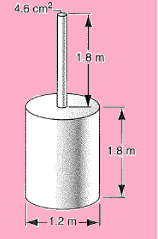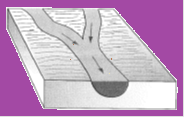Click to Chat

1800-1023-196

+91-120-4616500

CART 0

• 0

MY CART (5)

Use Coupon: CART20 and get 20% off on all online Study Material

ITEM
DETAILS
MRP
DISCOUNT
FINAL PRICE
Total Price: Rs.

There are no items in this cart.
Continue Shopping• Complete JEE Main/Advanced Course and Test Series
• OFFERED PRICE: Rs. 15,900
• View Details

```Solved Examples on Liquid at Rest:-

Question 1:-

A thin film of water of thickness 80.0 pm is sandwiched between two glass plates and forms a circular patch of radius 12.0 cm. Calculate the normal force needed to separate the plates if the surface tension of water is 0.072 N/m.

Concept:-

Surface tension is the surface force per unit length over which it acts.

The circumference of the thin films of circular patch of radius r between the glass plates is

L = 2πr

The surface tension acting on the thin films between the glass plates is

γ = F/L

Substitute L = 2πr in the above equation, the surface tension becomes

γ = F/2πr

Solution:-

From the above equation, the normal force needed to separate the plates is

F = 2πrγ

To obtain the normal force, substitute 12.0 cm for the radius r of the thin circular patch,0.072 N/m  for the surface tension of the water in the equation F = 2πrγ,

F = 2πrγ

= 2(3.14) (12.0 cm) (10-2 m/1 cm) (0.072 N/m)

= 5.42592×10-2 N

Rounding off to three significant figures, the normal force needed to separate the plates is 5.43×10-2 N.

_________________________________________________________________________________

Question 2:-

A cylindrical barrel has a narrow tube fixed to the top, as shown with dimensions in below figure. The vessel is filled with water to the top of the tube. Calculate the ratio of the hydrostatic force exerted on the bottom of the barrel to the weight of the water contained inside. Why is the ratio not equal to one? (Ignore the presence of the atmosphere.)Concept:-

The pressure at the bottom of the cylindrical barrel is

p = ρgh

Here, density of the liquid is ρ, acceleration due to gravity is g and height of the cylindrical barrel is h.

The hydrostatic force acting at the bottom of the barrel is

F = pA

Here, surface area of the barrel at the bottom is A.

The weight of the liquid is

W = ρgV

Here, total volume of the cylindrical barrel is V.

Solution:-

Now, the total height of the cylindrical barrel is

h = 1.8 m + 1.8 m

= 3.6 m

From above figure given in the problem, substitute 3.6 m for h in the equation p = ρgh gives

p = ρgh

= ρg (3.6 m)

This gives the pressure at the bottom of the barrel.

The volume of the thin barrel at the top is

V1 = (4.6 cm2) (1.8 m)

= (4.6 cm2) (10-2 m/1 cm)2 (1.8 m)

= 8.28×10-4 m3

The radius of the barrel at the lower part is

r = 1.2 m/2

= 0.6 m

The volume of the barrel at the lower part is

V2 = πr2 (1.8 m)

= (3.14) (0.6 m)2 (1.8 m)

= 2.03472 m3

Now, the total volume of the cylindrical barrel is

V = V1+V2

= 8.28×10-4 m3 + 2.03472 m3

= 2.035548 m3

The surface area of the barrel at the bottom is

A = πr2

= (3.14) (0.6 m)2

= 1.1304 m2

To obtain the hydrostatic force exerted by the liquid at the bottom, substitute ρg(3.6 m) for p  and 1.1304 m2 for A in the equation F = pA gives

F = pA

= ρg (3.6 m) (1.1304 m2)

= ρg (4.06944 m3)

To obtain the weight of the liquid in the cylindrical barrel, substitute 2.035548 m3 for V in the equation W = ρgV gives,

W =ρgV

= ρg (2.035548 m3)

Now, the ratio of the hydrostatic force to the weight of the liquid is

F/W = ρg (4.06944 m3)/ ρg (2.035548 m3)

= 1.9992

Rounding off to two significant figures, the ratio of the hydrostatic force to the weight of the liquid is 2.0.

The hydrostatic pressure is depending on the height of the liquid column. So, the same amount of liquid when taken in different volume of containers having different heights will not be the same. Weight is the volume times the density of the liquid. Therefore, the ratio is not equal to one.

_________________________________________________________________________________________

Question 3:-

Below figure shows the confluence of two streams to form a river. One stream has a width of 8.2 m, depth of 3.4 m, and current speed of 2.3 m/s. The other stream is 6.8 m wide, 3.2 m deep, and flows at 2.6 m/s. The width of the river is 10.7 m and the current speed is 2.9 m/s. What is its depth?Concept:-

The volume flow rate of the main river is equal to the sum of the volume flow rates of the two streams.

Assume that the cross-sectional area of the streams and the main river is rectangular in shape. The area of the streams is equal to the width of the streams times the depth of the streams. The area of the main river is equal to the width of the main river times the depth of the main river.

Solution:-

The cross sectional area of the first stream is

A1 = x1d1

Here, width of the first stream is x1 and depth of the stream is d1.

The volume flow rate of the first stream is

R1 = A1v1

= x1d1v1

Here, speed of the first stream is v1.

Similarly, the volume rate flow of the second stream is

R2 = x2d2v2

Here, width of the second stream is x2, speed of the water in the second stream is v2 and depth of the second stream is d2.

Now, the volume flow rate of the main river is

R3 = x3d3v3

Here, width of the main river is x3, speed of the water in the main river is v3 and depth of the main river is d3.

From the equation of continuity, the volume flow rate of the main river is equal to the sum of the volume flow rates of the two streams. It is given as

R3 = R1+R2

x3d3v3 = x1d1v1+ x2d2v2

d3 = [x1d1v1+ x2d2v2]/[ x3v3]

This gives the depth of the main river.

Substitute 8.2 m for x1, 3.4 m for d1, 2.3 m/s  for v1, 6.8 m for x2, 3.2 m for d2, 2.6 m/s  for v2, 10.7 m for x3 and 2.9 m/s for v3 in the above equation gives,

d3 = [x1d1v1+ x2d2v2]/[ x3v3]

= [(8.2 m) (3.4 m) (2.3 m/s) + (6.8 m) (3.2 m) (2.6 m/s)]/[(10.7 m) (2.9 m/s)]

= [(64.124 m3/s) + (56.576 m3/s)]/[31.03 m2/s]

= 3.8898 m

Rounding off to two significant figures, the depth of the main river is 3.9 m.

```### Course Features

• 728 Video Lectures
• Revision Notes
• Previous Year Papers
• Mind Map
• Study Planner
• NCERT Solutions
• Discussion Forum
• Test paper with Video Solution